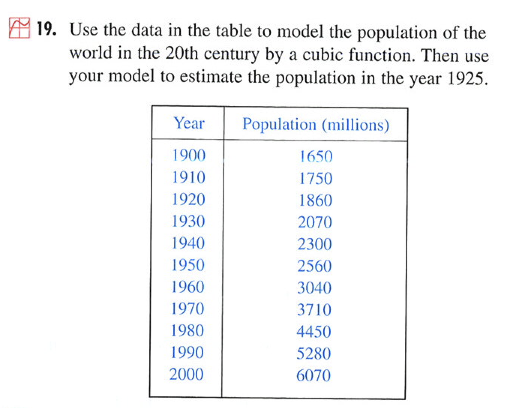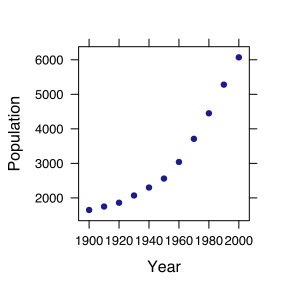# A Fitting Problem

A problem from Stewart Calculus: Concepts and Contexts 2/e p. 38. It appears in Chapter 1.• Why use “estimate” rather than “interpolate”?
• What are the essential properties of the function needed to create a reasonable interpolation?
• What's the point of using a cubic?
• How would you decide whether to use a cubic or some other function?

## Using mosaic

• Start R
• Load the package (you need do this only at the start of a session)
require(mosaic)

• Read in the data and display a bit
pop = fetchData("PREP-Stewart-World-Population.csv")

## Retrieving from
## http://www.mosaic-web.org/go/datasets/PREP-Stewart-World-Population.csv

head(pop)

##   Year Population
## 1 1900       1650
## 2 1910       1750
## 3 1920       1860
## 4 1930       2070
## 5 1940       2300
## 6 1950       2560


What skills are needed to do this? Careful spelling, attention to punctuation, use of quotes, understanding the structure of a file name, understanding the syntax for use of R functions, understanding assignment.

• Plot the data
plotPoints(Population ~ Year, data = pop)What do you need to know to answer the questions posed earlier?

Some techniques:

quadf = fitModel(Population ~ a * Year^2 + b * Year + c, data = pop)

##  1878

quadfResids = with(pop, Population - quadf(Year))


#### Fit a cubic

You figure it out!

#### Fit a Spline

splinef = spliner(Population ~ Year, data = pop)
splinef(1925)

##  1956

splinefResids = with(pop, Population - splinef(Year))


You can also try a monotonic spline. Use help(spliner) to find out how.

#### Fit an Exponential

This is hard, for reasons that relate to the data and numerics. Here, a guess is being made for a doubling time of 30 years.

expf = fitModel(Population ~ A + B * 2^((Year - 1900)/30), data = pop)


According to this model, what's the population in 1925?

### QUESTIONS

• Why are there so many extra parameters in the functions? Why not just a*Year^3 for the cubic?
• Which function is right?
• How well do the various functions work for extrapolation? Look up the world population in 2010 and check. Also, look up the world population in 1500 and check.
• What factors might influence world population that might mean that the rules of growth in 2000 might be different than 1900? If the system is changing, why can a mathematical function that doesn't change in form over the years capture the dynamics of population?
• Suppose that your job is to predict the world population in 2020. How would you build a model for this purpose?